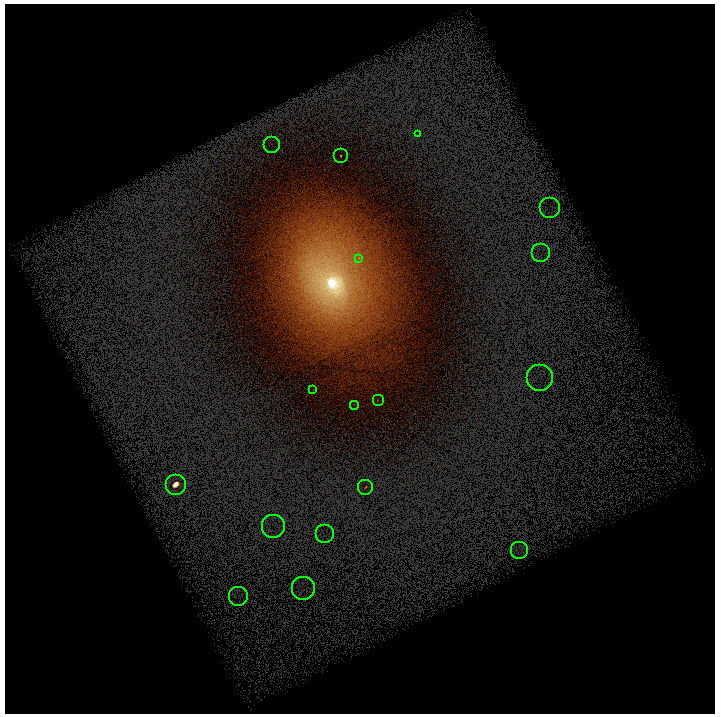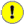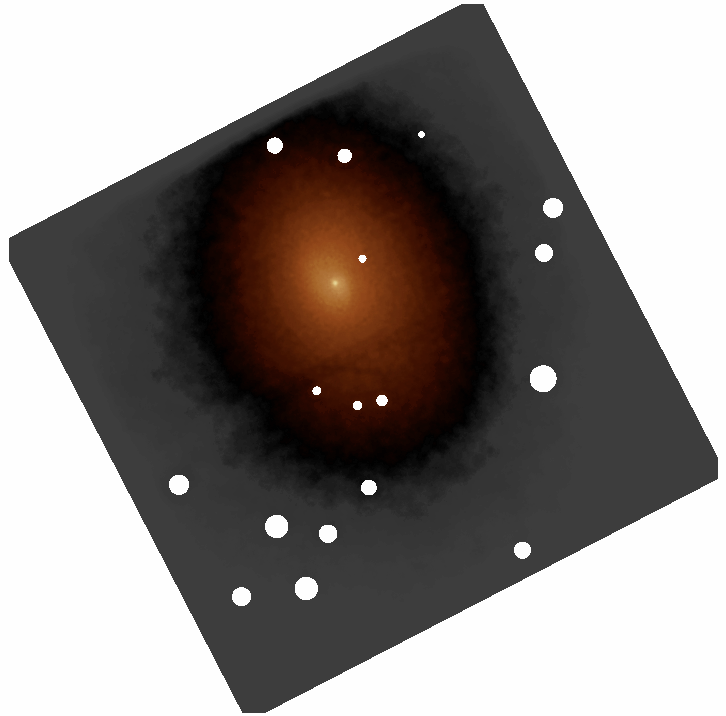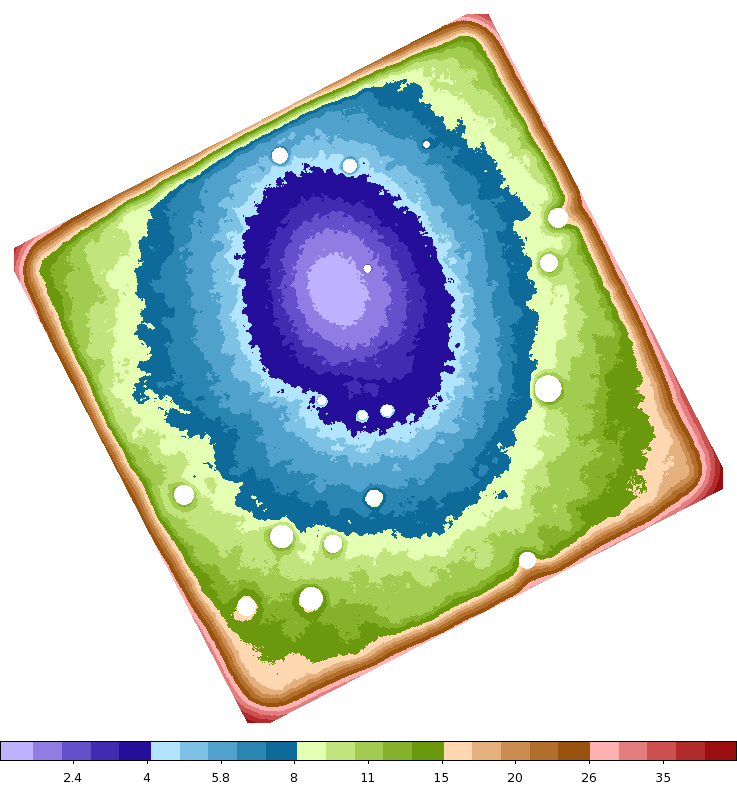# Find regions with minimum number of counts## Overview

#### Synopsis:

Certain algorithms work best when there is at least a fixed number of counts in the input. For example both spectral fitting and timing analysis are more robust when there are, for example, al least ~100 counts in the input.

For a single point in an image, one can determine the size of a circle that encloses at least a fixed number of counts by trial-and-error in just a few iterations. However, if the process is to be repeated for every pixel in an image, then more automated ways need to be used.

This thread shows how to use the dmimgadapt tool to determine the radius of a circle (or side length of a box) that encloses at least a fixed number of counts for every pixel in the input image.

#### Purpose:

Determine the size of circle that encloses at least a fixed number of counts for every pixel in input image.

Last Update: 23 Dec 2014 - Review for CIAO 4.7; minor edits only.

## Getting Started

`unix% download_chandra_obsid 4977 `

This thread will focus on the Abell 2029 cluster. Since the cluster is almost entirely imaged on ACIS-7, only that chip will be used to make an image using the fluximage script, after the data have recalibrated with chandra_repro.

`unix% fluximage "acisf04977_repro_evt2.fits[ccd_id=7]" abell2029 binsize=2 `

Finally, to improve speed we want to filter the image by the Field of View file. This will allow the subsequent tools to know which pixels are off the detector and therefore should not be considered in the analysis. The same ccd_id=7 filter is used with the field of view file as before. Note the [opt full] option is used to force the output image to stay the same size as the input; the default behavior of the CXC Datamodel is to shrink the image to the size of the filtered region. The data are shown in Figure 1.

```unix% dmcopy "abell2029_broad_thresh.img[sky=region(acisf04977_000N005_fov1.fits[ccd_id=7])][opt full]" img_with_fov
```

### Figure 1: Broad Band counts of Abell 2029, ObsID 4977### Figure 1: Broad Band counts of Abell 2029, ObsID 4977

The broad-band (0.5 to 7.0keV) counts of Abell 2029 from ObsID 4977, with data restricted to just ACIS-7 (ACIS-S3). Several point sources have been identified and drawn as green circles. They will be excluded from the analysis below. Data are binned by a factor of 2 (1 pixel=~1'').

## Determine the radius of a circle that encloses constant counts

For this example, the goal is to find the radii of circles that enclose at least 250 total counts. As shown in Figure 1 there are several point-like sources that need to be excluded from the analysis. They are listed below as saved in a CIAO format ASCII region file:

```unix% cat ciao.reg
# Region file format: CIAO version 1.0
circle(3864.5183,3571.0756,20.410863)
circle(3989.5161,3347.0484,19.129071)
circle(4118.9925,3363.5201,23.330288)
circle(4244.2028,3565.3626,15.837317)
circle(4551.4603,3439.9762,17.17467)
circle(4060.042,3487.4618,23.425518)
circle(4195.866,4228.868,14.400337)
circle(4231.571,4023.179,8.1002516)
circle(4594.5131,4034.4216,18.159209)
circle(4612.705,4124.623,20.036573)
circle(4056.321,4249.4199,16.258138)
circle(4140.3128,3758.8112,8.9226531)
circle(4221.6053,3729.3411,9.6319044)
circle(4270.4822,3739.5,11.518549)
circle(4592.764,3782.988,26.945645)
circle(4162.764,3472.988,18.490854)
circle(4349.5,4271.5,6.4448495)
```

The dmimgadapt tool can now be used to determine the radius of a circle that enclose at least 250 counts at each point in the image. The key is to select the tophat convolution kernel function. The tophat function is exactly a circular shape kernel with constant amplitude. The size of the tophat will increase until the number of counts is met or the maximum radius limit is hit.Note

If for some reason square regions were a more natural shape for the data, then the same technique can be used using the box convolution kernel function. Note that the output is the total side-length rather than the radius.

In this example, the tool will only consider radii from 1 pixel to 50 pixels in 0.5 pixel increments (giving a total of numrad=99 linearly spaced radii). Different datasets may require adjusting these parameters: this should be done carefully as the execution time of the program may slow down considerably with many or very large radii.

```unix% pset dmimgadapt infile="img_with_fov[exclude sky=region(ciao.reg)]"
Pre-computing convolution kernels
First iteration: determine scales and normalization
Percent complete: 99.86%
Second iteration: computing final normalized values
```

Giving the binning factor (2) and since the field of view was explicitly included in the image, this should only take a few 10's of seconds to run. Two output files are created:

smoothed_image

is the adaptively smoothed image, which is typically the main output file. It is shown in Figure 2. The values in the excluded region and off the detector have not been smoothed and have been set to NaN (shown white).

An image whose pixel values are the radius of a circle that encloses at least 250 counts, shown in Figure 3. The radii go from 1 pixel in the center of the image to around 15 pixels at the edge of the detector (more at the very far extreme). Again the off-chip and excludeds pixel have the radii set to NaN as they did not contribute to the calculation.Important

The radii are in logical (or image) pixels. The images where created with binsize=2 which will need to be taken into account whenever the radii file are used.

Care should be taken at the edge of the image where the minimum number of counts may not have been achieved given the maxrad radius. The dmimgadapt sumfile parameter can also be set and used to locate parts of the image where number of counts fell below the desired level.The broad band image of Abell 2029 adaptively smoothed using a tophat kernel that varies between 1 and 50 pixel in 0.5 pixel increments.

Pixels outside the field of view are set to NaN, as are the point sources that were excluded.

### Figure 3: Radii that Yield Minimum of 250 counts### Figure 3: Radii that Yield Minimum of 250 counts

This is a map of the radius of a circular tophat needed to obtain at least 250 counts at each point in the image. At the center of the cluster, the radius is 1 pixel (minimum value) and increases in size up to approximately 15 pixels at the edge of the detector.

The pixels values are the radii expressed in logical/image pixel size. When the radii are used, the original binsize (2, in this example) will need to be taken into account.

## How to use

The output radii_min_250_counts image can now be used like any other image file. The image values can be retrieved and used to do simple analysis. For example:

```unix% punlearn dmcoords
unix% dmcoords radii_min_250_counts asol= op=cel ra=15:11:00.049 dec=+5:42:26.00 celfmt=hms verb=0
unix% pget dmcoords x y
4057.50599965482
3699.505054973268

unix% dmstat abell2029_broad_thresh.img"[sky=circle(4057.50599965482,3699.505054973268,11)]" cen- sig- med-
EVENTS_IMAGE
min:	0 	      @:	( 4055.5 3693.5 )
max:	8 	      @:	( 4051.5 3691.5 )
mean:	2.9072164948
sum:282
good:	97
null:	47
```

Here we have located a specific RA,Dec position in physical coordinates using dmcoords; used evalpos to extract the radius at that location, and then run dmstat using a circle at that location with a radius, scaled by the binsize (5.5*2 = 11), retrieved from the radius image.

A more advanced example is to write a Python script that loops over all the pixel in the image and performs a given task. An illustrative example is provided here:

```from pycrates import read_file
from ciao_contrib.runtool import dmstat
import numpy as np

binsize=2.0

img_data = img.get_image().values
xlen = len(img_data)
ylen = len(img_data)

sky = img.get_transform("sky")

for jj in range(ylen):
for ii in range(xlen):
# Skip edges
if np.isnan(img_data[jj,ii]) or img_data[jj,ii]>=15:
continue
xy = sky.apply( [[ii+1.0,jj+1.0]] )
region = "circle({},{},{})".format( xy, xy, binsize*img_data[jj,ii])
centroid=False, sigma=False, median=False )
```

While it may not be too interesting to run dmstat for each region, that command could be replaced with glvary to get variability information for each region or even specextract to extract and fit a spectrum.

## Summary

This thread has shown how to use dmimgadapt with a tophat convolution kernel function to determine the size of a circular region needed to enclose a fixed number of counts in an image.

It has also shown how a user can exclude regions (eg point sources) and include the field of view to limit the data the tool needs to operate on.

The result of this thread is an image whose pixels are the radius of the circle needed to enclose the specified number of counts, in image/logical pixels. The radii need to be scaled by the binsize when using them in physical coordinates.

## History

 31 Mar 2014 Initial version. 23 Dec 2014 Review for CIAO 4.7; minor edits only.